# Hoang, Chinh T.

## 2-colouring a graph without a monochromatic maximum clique ★★

Author(s): Hoang; McDiarmid

Conjecture   Ifis a non-empty graph containing no induced odd cycle of length at least, then there is a-vertex colouring ofin which no maximum clique is monochromatic.

Keywords: maximum clique; Partitioning

## Hoàng-Reed Conjecture ★★★

Author(s): Hoang; Reed

Conjecture   Every digraph in which each vertex has outdegree at leastcontainsdirected cycles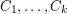such thatmeetsin at most one vertex,.

Keywords:

## The stubborn list partition problem ★★

Author(s): Cameron; Eschen; Hoang; Sritharan

Problem   Does there exist a polynomial time algorithm which takes as input a graphand for every vertex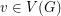a subset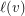of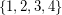, and decides if there exists a partition ofinto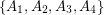so that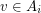only ifand so thatare independent,is a clique, and there are no edges betweenand?

Keywords: list partition; polynomial algorithm